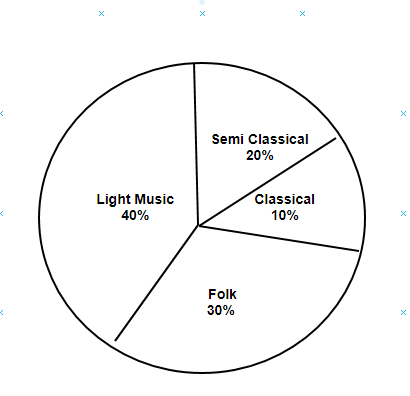# Ex.5.2 Q1 Data Handling Solutions - NCERT Maths Class 8

Go back to  'Ex.5.2'

## Question

A survey was made to find the type of music that a certain group of young people liked in a city. Adjoining pie chart shows the findings of this survey.

From this pie chart, answer the following:

(i) If $$20$$ people liked classical music, how many young people were surveyed?

(ii) Which type of music is liked by the maximum number of people?

(iii) If a cassette company were to make $$1000$$ CD’s, how many of each type would they make?Video Solution
Ex 5.1 | Question 1

## Text Solution

What is known?

Pie chart showing the distribution of music.

What is unknown?

(i) The number of young people were surveyed if $$20$$ people liked classical music

(ii) The type of music is liked by the maximum number of people

(iii) Each type of cassettes would they make, if a cassette company were to make $$1000$$ CD’s

Reasoning:

A circle graph or pie chart shows the relationship between a whole and its parts.

Steps:

(i) $$10\%$$ represents $$20$$ people.

Therefore, $$10\%$$ represents

\begin{align}= \frac{{100 \times 20}}{{10}} = 200\end{align} People

Hence, $$200$$ young people were surveyed.

(ii)  From the pie chart,it can be easily observed that the light music is represented by the maximum part of the pie chart $$($$i.e $$40 \%)$$Hence,light music is liked by the maximum number of people.

(iii)Number of CD’s of classical music

\begin{align}= \frac{{10 \times 1000}}{{100}} = 100\end{align}

Number of CD’s of semi-classical music

\begin{align}= \frac{{20 \times 1000}}{{100}} = 200\end{align}

Number of CD’s of light music

\begin{align}= \frac{{40 \times 1000}}{{100}} = 400\end{align}

Number of CD’s of folk music

\begin{align}= \frac{{30 \times 1000}}{{100}} = 300\end{align}

Video Solution
Data Handling
Ex 5.2 | Question 1

Learn from the best math teachers and top your exams

• Live one on one classroom and doubt clearing
• Practice worksheets in and after class for conceptual clarity
• Personalized curriculum to keep up with school